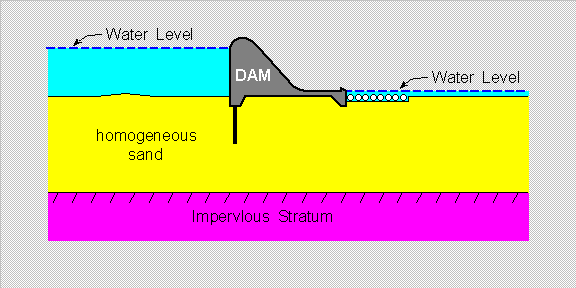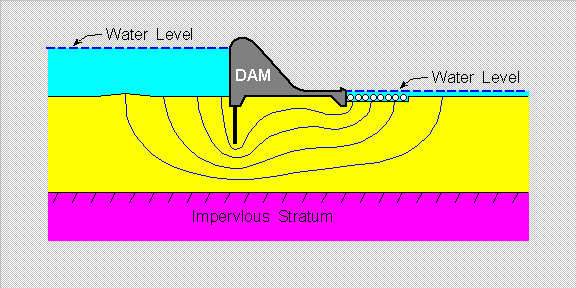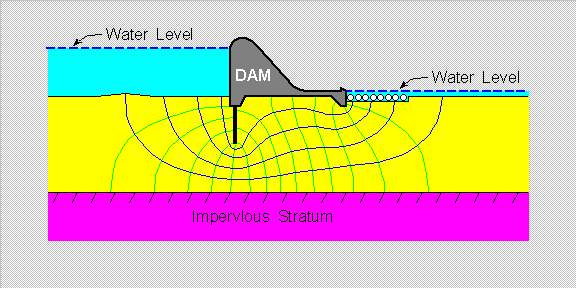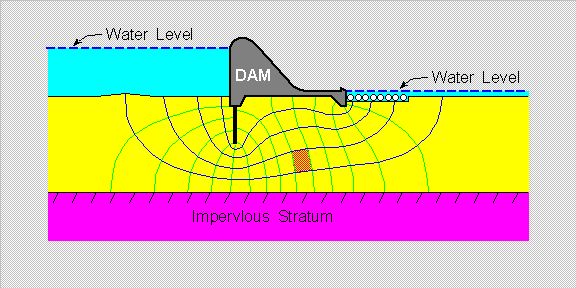Flow Nets

# An Approach to the Mechanics of Fluids

## Section VI - { Flow Net } in Soil Mechanics

### Summary for the Section :

• In this Section , we shall present the concept of { Flow Net } ,

• a quasi-empirical method from { Soil Mechanics } .

• This then give rise to the notion of :

• using the { General-Random-Type Six-Face Object } as the [ finite element ] in { Fluid Mechanics Analysis } .

### { Flow Net } in Soil Mechanics :

• { Flow Net } is simply a quasi-empirical method used in { Soil Mechanics } :

• Let us look , for a brief moment , at the seepage of water under a Dam , as per this diagram below :• The { Flow Net } method is then as follows :

• First , we draw-in , roughly what we think are the { Stream-Lines } for the flow :

• as marked by the { blue-color lines } in the diagram below .• Next , we draw-in , lines that intersect the { Stream-Lines } at [ Right-Angles ] :

• as marked by the { green-color lines } in the diagram below .

( Right-Angles ] are [ 90-degrees angles ] )• If the { intersection angles } do not look-like [ Right-Angles ] ,

• we simply make adjustments to the { blue-color lines } and the { green-color lines } :

• until the { intersection angles } do look-like [ Right-Angles ] .

• We shall not go into the full details of the { Flow-Net } method , but will simply note here :

• that each { Finite Element } in the { Flow Net } is a { planar surface } with 4 [ nodal-points ] ,as marked in { orange-color } in the diagram above .

( see [ Soil Mechanics in Engineering Practice - Karl Terzaghi and Ralph B. Peck ] - ISBN 0-471-85273-2 for full details . )

### The { General-Random-Type Six-Face Object } as a [ Finite Element ] :

• We simply note here that with 4 { nodal-points } , we can always form :

• a planar [ Finite Element ] with 4 [ straight-edges ] ,

• which is essentially a { quadrangle } .

• When we extend this to { 3-D Space } , we can always use :

• the { General-Random-Type Six-Face Object } as the [ Finite Element ] ,

• which has 8 { nodal-points } and exactly 6 [ faces ] which are all [ planar surfaces ] .

We then have a clear and distinct advantage here :

• each [ face ] of the [ finite element ] here is always in { full contact } with the corresponding { face } of the adjacent [ finite element ] ,

• so that there are no [ gaps ] in-between and unbroken { continuity } can always be achieved and maintained , if so desired .

#### go to Top Of Page

• Next , we shall look at the usage of this type of [ finite elements ] in a { steady flow } situation .